Learning Objective

In this lesson we will learn how Punnett squares can be used to predict genotypes and phenotypes of offspring.

Learning Outcomes

By the end of this lesson you will be able to:

• Complete a Punnett square to determine the possible genotypes and phenotypes of offspring for:
• Dominant and recessive inheritance
• Incomplete dominance and codominance
• State the probability of particular genotypes or phenotypes in offspring, based on a Punnett square.
• Explain why observed phenotype ratios can differ from predicted phenotype ratios.

Click images to preview the worksheets for this lesson and the Year 10 Biology Workbook.

## Introduction to Punnett Squares

• To observe the transmission of alleles from parents to offspring, biologists use diagrams called Punnett squares.
• By using Punnett squares, the possible genotypes and phenotypes of offspring can be determined, as well as their probability of occurring.
• Punnett squares can be used to explain dominance, incomplete dominance, codominance and sex-linked inheritance.
• Punnett squares consist of a larger square divided into four smaller squares, in a 2×2 format.
• The alleles of parents are written above and beside the Punnett square and the genotypes of offspring are written inside the Punnett square.
• The parental alleles correspond with the possible gametes produced during meiosis.
• For homozygous individuals, only one type of allele is possible.
• For heterozygous individuals, two types of alleles are equally likely.
• The possible genotypes of offspring represent fertilisation events between particular allele pairings.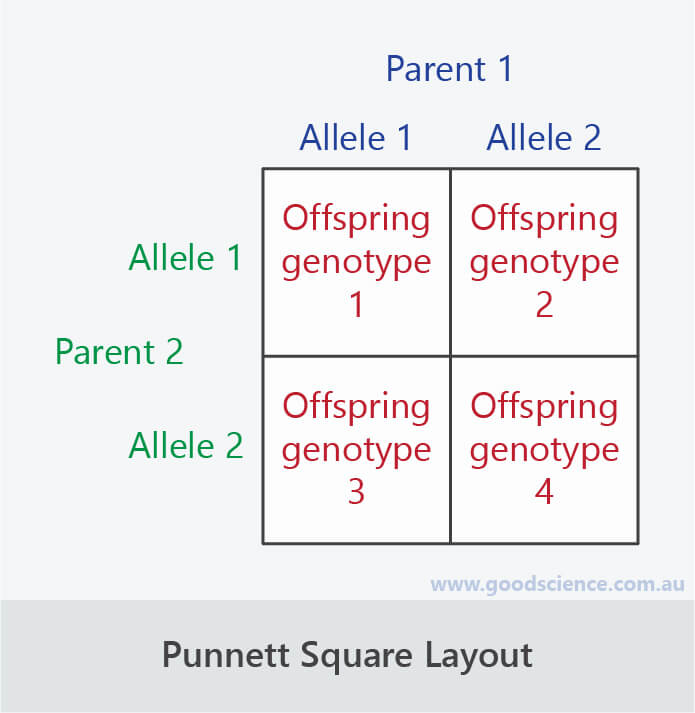The general layout of a Punnett square

## Steps for Completing a Punnett Square (Dominant and Recessive Inheritance)

• To illustrate how to use a Punnett square, we will use an example looking at body colour in fruit flies.
• The allele for tan body colour (T) is dominant over the allele for ebony body colour (t).
• Consider a mating between two flies – one with the genotype Tt and the other with the genotype tt.
• The individual with the Tt genotype will have tan body colour and the individual with the tt genotype will have ebony body colour.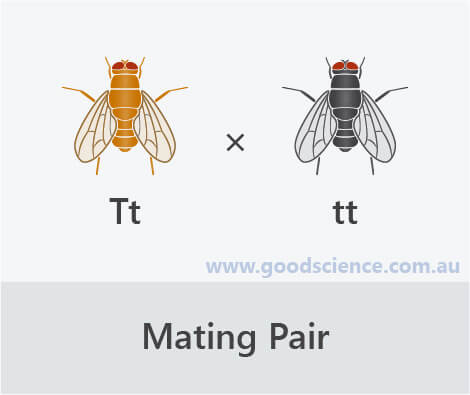### Step 1: Parental Alleles

• Write the two alleles of one parent above the Punnett square and the two alleles of the other parent to the left of the Punnett square.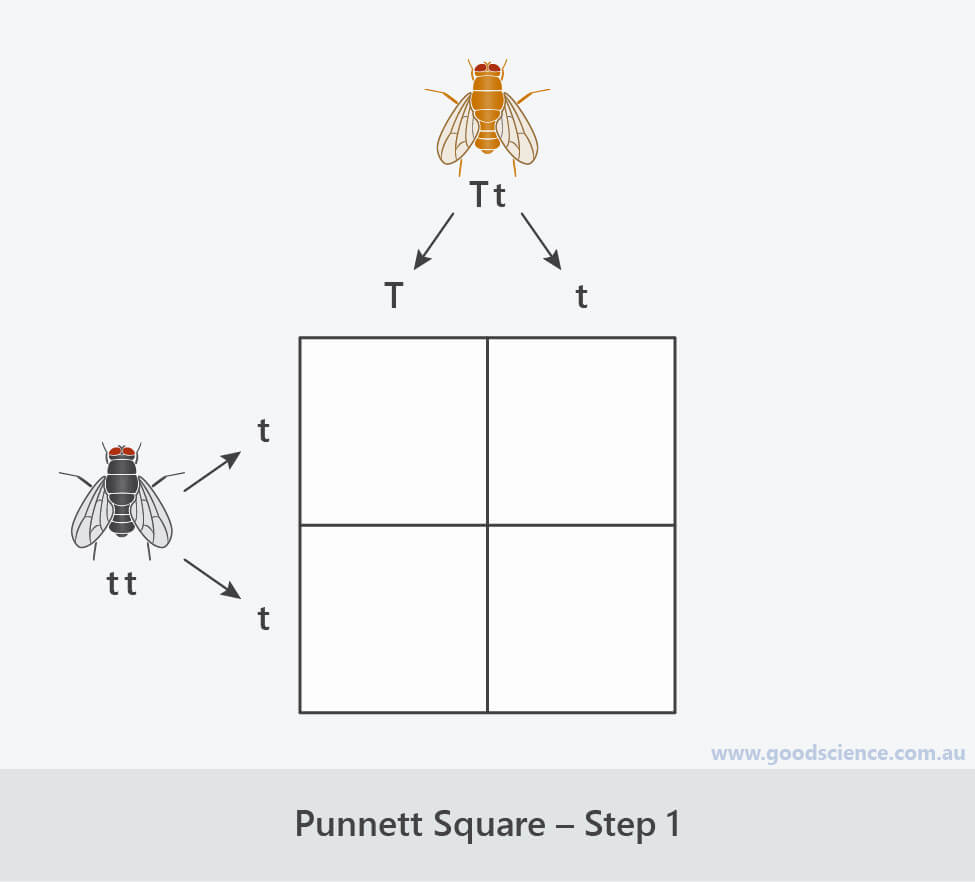Step 1: Write the parental alleles.

### Step 2: Offspring Genotypes

• Determine the genotypes of offspring resulting from each parental allele combination in the four squares.
• Always write uppercase letters (dominant alleles) before lowercase letters (recessive alleles).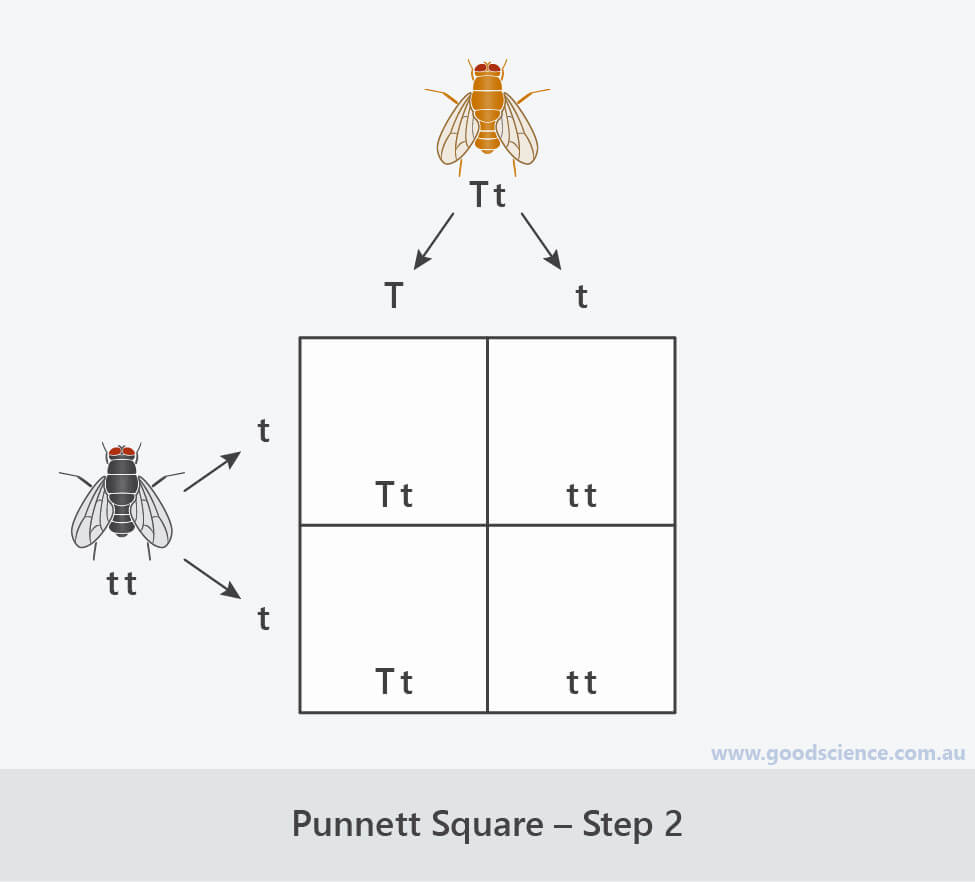Step 2: Determine the genotypes of offspring.

### Step 3: Offspring Phenotypes

• Determine the phenotypes of offspring corresponding to each genotype.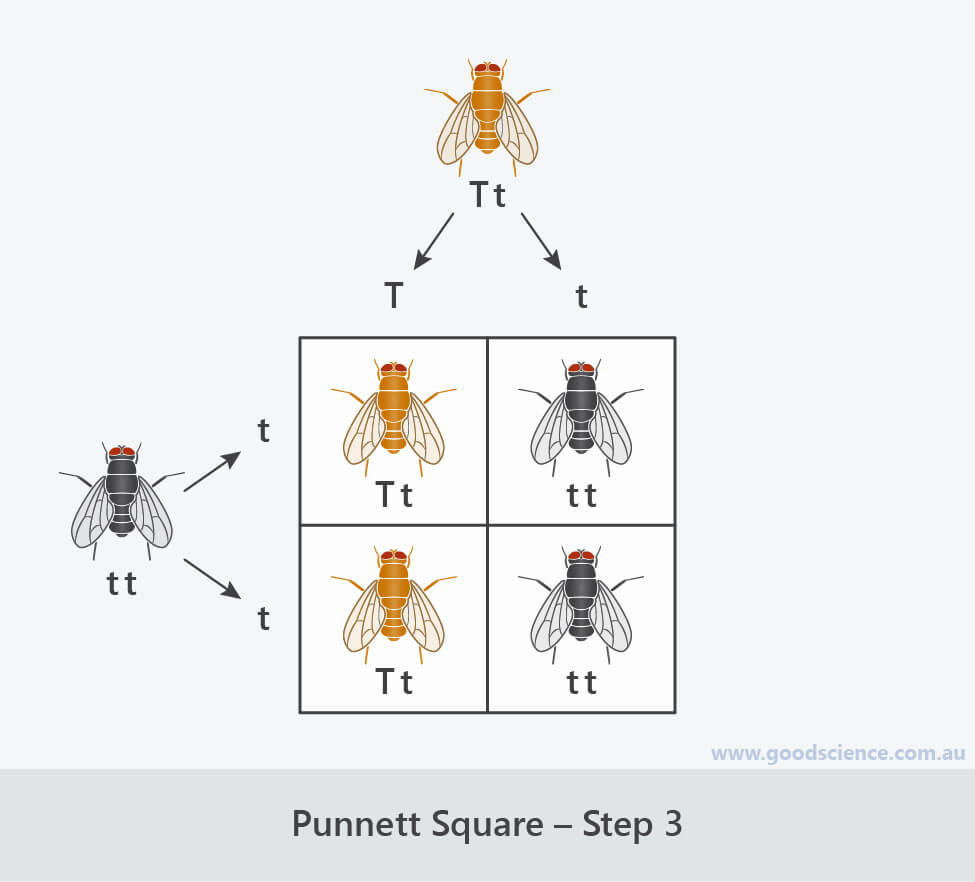Step 3: Determine the phenotypes of offspring.

### Step 4: Probability Calculations

• Determine the probability of each genotype and phenotype occurring in the offspring.
• Each square represents a 25% probability. If a genotype or phenotype occurs in more than one square, add the probabilities together.
• In this example:
• There is a 50% probability of offspring having the Tt genotype and a 50% probability of offspring having the tt genotype.
• There is a 50% probability of offspring having the tan phenotype and a 50% probability of offspring having the ebony phenotype.
• Note, in this example the genotype and phenotype ratios are the same, but this will not be the case if there are homozygous dominant and heterozygous offspring.
• Remember that Punnett squares determine probabilities, not actual outcomes. It is possible that the pair of flies above could produce more tan flies than ebony flies, or vice versa.
• However, the larger the number of offspring produced, the less the influence of chance affecting outcomes; therefore, the greater the likelihood of observed ratios matching predicted ratios.
• The only times Punnett squares predict exact outcomes is when there is either a 0% or 100% probability. For example, there is a 0% chance of homozygous tan flies resulting from this mating.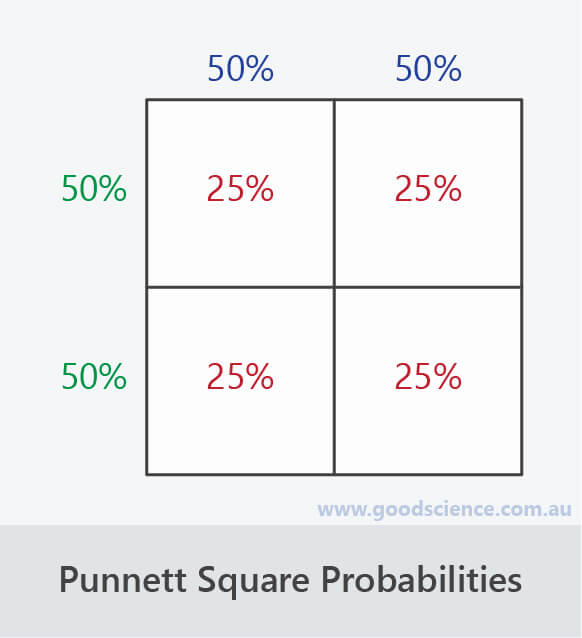The probability of each parental allele is 50%
Therefore, the probability of each offspring genotype is 25%

## Punnett Squares for Incomplete Dominance and Codominance

• The procedure for completing Punnett squares for traits that exhibit incomplete dominance or codominance is the same as for dominance.
• The only difference is how alleles are written (two different capital letters) and how phenotypes are determined from genotypes.
• The Punnett squares below shows hypothetical examples of incomplete dominance and codominance for flower colour, where R is the allele for red flowers and W is the allele for white flowers.Punnett squares for incomplete dominance and codominance are the same as those for dominance.

## Punnett Squares for Sex-Linked Traits

• The procedure for completing Punnett squares for sex-linked traits is also the same as for dominance, except for one key difference: the probability of each genotype and phenotype in the offspring needs to be determined separately for males and females.
• The Punnett square below shows the inheritance of eye colour in fruit flies, which is X-linked. The allele for red eyes (R) is dominant over the allele for white eyes (r).For sex-linked traits, probabilities need to be determined separately for males and females.

• In this example:
• There is a 50% probability of female offspring having the XRXR genotype and a 50% probability of female offspring having the XRXr genotype.
• There is a 50% probability of male offspring having the XRY genotype and a 50% probability of male offspring having the XrY genotype.
• There is a 100% probability of female offspring having the red eye phenotype.
• There is a 50% probability of male offspring having the red eye phenotype and a 50% probability of male offspring having the white eye phenotype.

## Summary

• Punnett squares are diagrammatic methods for determining the possible genotypes and phenotypes of offspring, as well as their probability of occurring.
• Punnett squares consist of a larger square divided into four smaller squares, in a 2×2 format.
• The alleles of parents are written above and beside the Punnett square and the possible genotypes of offspring are written inside each smaller square.
• Each smaller square represents a 25% probability.
• The steps for completing a Punnett square are similar for all modes of inheritance:
• Write the two alleles of one parent above the Punnett square and the two alleles of the other parent to the left of the Punnett square.
• Determine the genotypes of offspring resulting from each parental allele combination in the four squares.
• Determine the phenotypes of offspring for each genotype.
• Determine the probability of each genotype and phenotype occurring in the offspring.
• Punnett squares determine probabilities, not actual outcomes.
• The larger the number of offspring produced, the greater the likelihood of observed ratios matching predicted ratios.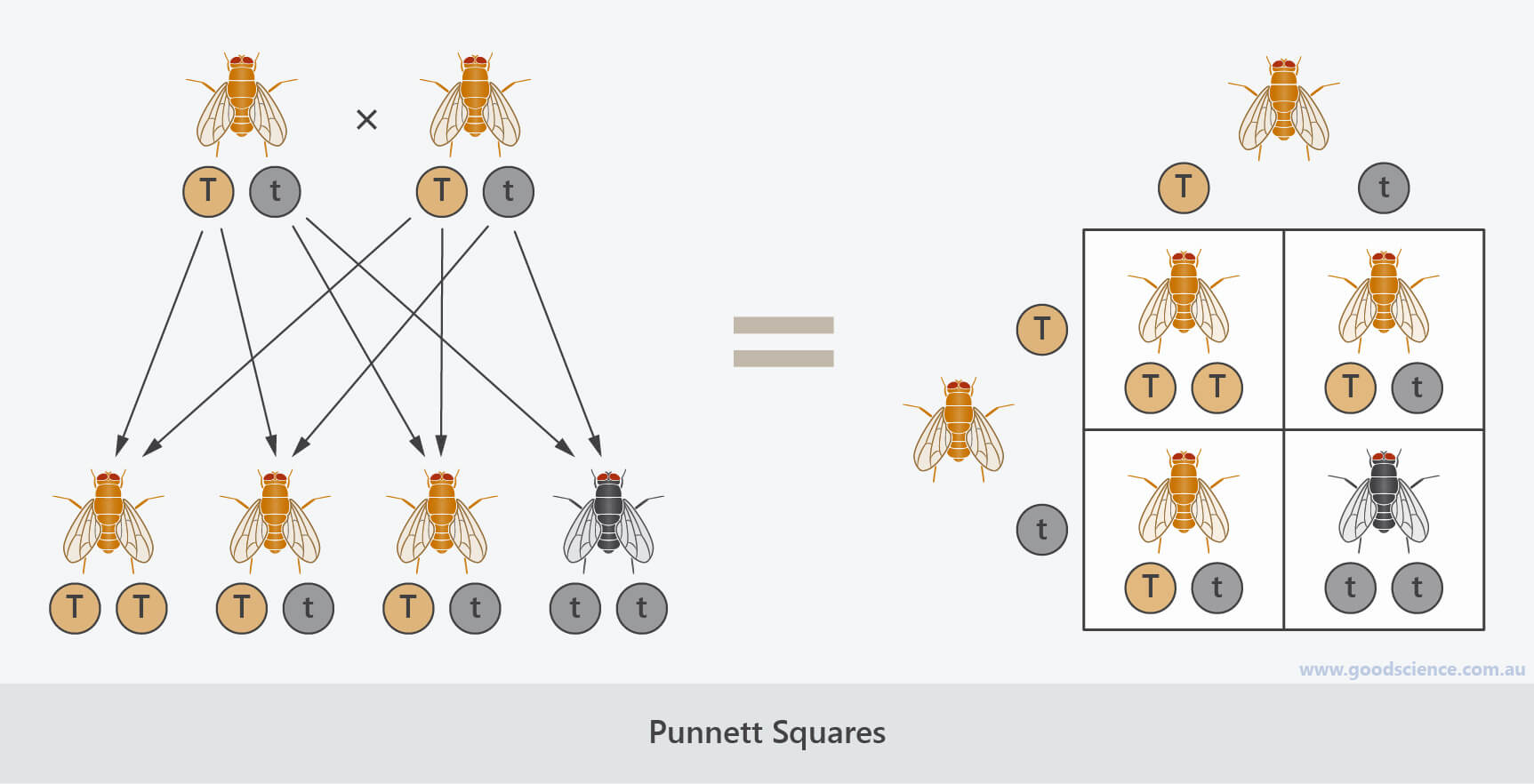Punnett squares are a simple way of determining the possible genotypes and phenotypes of offspring.